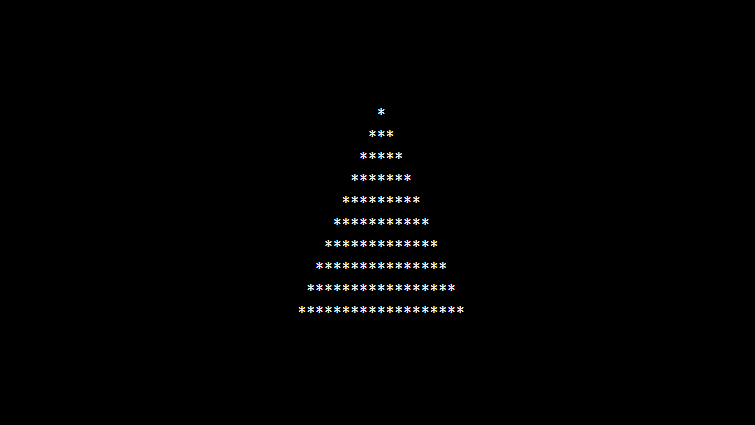# PHP Program to Print Pyramid

In this article write a PHP Program to Print Pyramid pattern. This Program first takes the numbers of rows and then prints pattern using nested for loops. This kind of problems are useful for beginners to understands the fundamentals of for loops and spaces.

## PHP program to Print Pyramid Pattern using * and loop.

```<?php
echo "<pre>";
\$space = 10;
for (\$i = 0; \$i <= 10; \$i++) {

for (\$k = 0; \$k < \$space; \$k++) {
echo "&nbsp;";
}
for (\$j = 0; \$j < 2 * \$i - 1; \$j++) {
echo "*";
}

\$space--;
echo "<br/>";
}
echo "</pre>";
?>
```

### Output:Explanation:

The program is used to build a pyramid using * (asterisk) and for that you have to understand the for loop which is implemented inside the program.

`\$i = 0 till 10`
```\$k = 0 till \$k < \$space
```
```echo "&nbsp;";
```
```\$j = 0 till \$j= 2 * \$i - 1
```

and inside that loop block prints the asterisk (*) symbol. Outside the inner for loop, you have to decrement the value of variable \$space by 1

`echo "<br/>"; `
1. Mukesh says

Great Examples!

1. Full Stack developer says

ohh thanks

2. y joy chandra singha says

awesome, and simple, and very clear to understand.

1. Full Stack developer says

I’m glad it helped you. Happy coding.

best example

1. Full Stack developer says

4. Holly Hettrick says

Fantastic data, Appreciate it!|

Alternative approach, building a multi dimensional array containing all the values of the pyramid.

<?php
\$n = 10;
\$pyramid = [];
for (\$i = 1; \$i < \$n; \$i++) {
\$pyramid[\$i] = array_pad([], \$n * 2 – 1, ' ');
\$middlePoint = floor((\$n * 2 – 1) / 2);
for (\$starIndex = \$middlePoint – (\$i – 1); \$starIndex <= \$middlePoint + (\$i – 1); \$starIndex++) {
\$pyramid[\$i][\$starIndex] = '*';
}
}

echo '’;
foreach (\$pyramid as \$row) {
echo implode(”, \$row).”\n”;
}
echo ”;